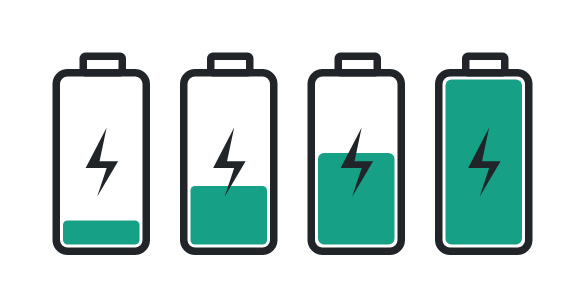# Battery Charge Time Calculator

Input battery capacity, charge rate current, & hit calculate button to find charge time using battery charge time calculator

Formula:
Time = Battery CapacityCharge Rate Current

Give Us Feedback

## Battery Charge Time Calculator

Battery Charge Time Calculator is used to estimate the time required to charge a battery. The tool takes the values of the capacity of the battery and the charging rate to calculate the estimated time to charge a battery.

## What is the battery charge time?

Battery charge time is the time that is required to charge a battery to its maximum capacity. The charge time of the battery is totally dependent on the charge rate current and battery capacity.Battery capacity

Battery capacity is the amount of energy that a battery can store, usually measured in ampere-hours (mAh).

Charging rate

The charging rate is the rate at which the battery can be charged, typically measured in amperes (mA).

## Formula of battery charge time

The formula for battery charge time is:

Time = battery capacity/charge rate current

## How to calculate battery charge time?

Here is an example to calculate battery charge time.

Example

Calculate the battery charge time if the capacity of the battery is 7000 mAh and the charging rate is 40 mA.

Solution

Step 1: Take the given data.

battery capacity = 7000 mAh

charge rate current = 40 mA

Step 2: Take the formula of battery charge time.

Time = battery capacity/charge rate current

Step 3: Put the values in the battery charge time formula.

Time = 7000/40 = 175 hours.

### Math Tools